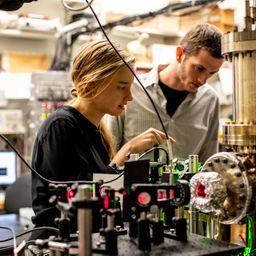## 5.7 \times 10^{3} \text { photons / second }

Quantum Physics

Atomic Physics

### Discussion

You must be signed in to discuss.
##### Top Physics 103 Educators##### Christina K.

Rutgers, The State University of New Jersey

LB##### Marshall S.

University of Washington##### Aspen F.

University of Sheffield

### Video Transcript

Okay, so in this problem we have, we know that we need a certain intensity in order to see. So let's write down that intensity. Um, in the dark, it's It's 4.0 times 10 to the minus 11 watts per meter squared. I know that's the intensity. I don't know if they actually stay that that quantity is an intensity. But any time, that's what per meter power over area. That's gonna be, um, intensity. And the wavelength is five times 10 of the two nanometers. So 500 nanometers? Yeah, I guess this intensity would depend on the way plank. So it's good that it's specified the wavelength that this intensity applies to. And, um so if the diameter of the eyes 8.5 millimeters, it's just just plain meters. Um, then we want to get, um, How many photons per second, Uh, under the eye. So it's gonna be interesting, eh? So let's just do everything for one second. And so So here's the I, um, and then here's some photons coming at the I. And so why has this certain area? We can figure out how many Watts need to be entering the eye by taking this intensity and multiplying by the the area. And we can get the area because we know the diameter. And so we can say that the radius then just put in terms of radius. It's a little more intuitive. The radius is this divided by two. And so, um, so we could get the power the throat entering the eye So the power is gonna be this I times a So it's gonna be I times pi r squared. And so this if we calculated than that, will give us the power. Um And then if we let's just consider a unit of one second, um so for one second, then you can the energy So is his powers energy over time. And if time is one, when power is equal to energy and magnitude and so after one second the energy is equal Thio I times pi r squared. Now that we know that the energy per photons is, we can find that using this So the energy per photo time a miss equal to each see over lambda That's just a formula. Um ages. Planck's constant C is the speed of light and so we won't know what we want to do is want to take this and divide. And we want to divide this by the energy per photons. So this is the total energy. And then, um, by by the energy per photo time, then we'll get the number of photons. So that's so then we want to evaluate this. So now we can say this is equal to the use this and then was that. But then, now that I added this divided by H C over Lambda. Now, this is the number of photons, and so I'm gonna pause while I plug this into a calculator. Okay, um, I evaluated this and I realized it was a mistake here. This shouldn't This one should be here. It should just be 4.0. So with that modification, um, I get this. This is equal to 5.7 times 10 to the third photons per second. Yeah. I mean, I could have done this, Not by being like okay, consider one second, but since I know it's linear, um, like Ieave, you know, if you double the amount of seconds, you double the amount of photons I could just sort of use this as a tool. If it's not letting her, you can't do that for kind of interesting. It's kind of a lot of photons that you would need, Um, likes. It seems like it to me, but I get maybe not. I mean, that's how many reactions you need. And given that usually and matter, there's another God rose number of particles. This doesn't really seem like a ton of reactions. Issue more so be limited by the light source, reflecting off of things you'd be trying to see.University of Washington

#### Topics

Quantum Physics

Atomic Physics

##### Top Physics 103 Educators##### Christina K.

Rutgers, The State University of New Jersey

LB##### Marshall S.

University of Washington##### Aspen F.

University of Sheffield